0 items

# The effect of some winding defects on the output of a Rogowski coil

## Part 1 - Basic theory and concepts

An ideal, perfectly-wound Rogowski coil should respond only to currents which thread the coil. It should not matter if the current-carrying conductor is off axis or threads the coil obliquely. Furthermore, the coil should have zero response to electric currents and other sources of magnetic fields which are external to the loop of the coil. These properties follow as a consequence of Ampere’s Law.

In a 'real' coil there are inevitably winding defects and the ideal properties described above cannot be achieved in practice. This Note describes a simple method for determining how winding defects can affect the performance of a coil. The method can be used for accurate calculations as well as providing a method for making qualitative estimates without the need for mathematical analysis.

For de-mountable coils, such as flexible coils and split-rigid coils, the most significant potential defect is the inevitable gap in the winding where the coil is opened so that it can be placed round the conductor. The method described for calculating the effect of winding defects, such as a gap, is illustrated by a number of examples and is compared with some actual measurements on coils.

Keywords: Rogowski coil, mutual inductance, measurement, Ampere’s law, electric current , pick-up, cross-talk, bus-bar

### List of symbols

Symbol Description
A cross-sectional area of a coil former
a radius of the coil cross-section
$\alpha$ angle between the direction of the winding and the magnetic field (Fig A1)
D width of a ‘Race-track’ coil (Fig 7)
E distance of an external bus-bar (Fig 7)
F height of a ‘Race-track’ coil (Fig 7)
$\phi$ magnetic flux
g winding gap
g% winding gap expressed as a percentage of the coil length
H magnetic field
I current being measured by the coil
L length of a section of flexible coil
M mutual inductance of coil
${\mu }_{0}$ permittivity of free space
n turns density (turns/m)
r distance of a section of coil from the conductor
S 'Strength' of a section of coil (Eq 7)
$\theta$ angular position of a section of coil (Fig 2)
T time
${V}_{coil}$ voltage output from a coil
W width of a bus-bar

### Introduction

Rogowski coils are now widely used for electric current measurements in many applications ranging from power quality monitoring of buildings to the measurement of lightning strikes, very large currents in arc furnaces and switchgear testing. They have several advantages over conventional current transformers. A well-made coil with a near-perfect winding can come close to the ideal for a current transducer in that it responds only to currents that thread the loop of the coil and is not sensitive to the distribution of currents within the loop. Furthermore, it will not respond to electric currents or other sources of magnetic field which are outside the loop of the coil.

A 'perfect' winding means that the cross-section of the coil former is exactly constant round the coil and the turns density of the winding is also exactly uniform. Although considerable efforts are made in the construction of coils it is inevitable that some imperfections will always remain. In this Note we provide a quantitative relationship between winding defects and the resulting measurement errors when the coil is used to measure the current in a conductor. The relationship can also be used to make an intuitive assessment of the effect of a winding defect without the need for mathematical analysis.

There may also be other current-carrying conductors in the vicinity of the coil but not threading it. These will cause 'pick-up' errors which depend on the size of the defect and the distance and orientation of the external conductors. This paper shows how the influence of external conductors can be determined.

The method used for calculating errors due to coil defects is illustrated by a number of examples which are based on simple geometrical concepts. Calculations will also be compared with actual measurements on coils with defects.

### 2. Coil Construction

The Rogowski coil is an ‘air cored’ toroidal winding placed round the conductor in such a way that the alternating magnetic field produced by the current induces a voltage in the coil [Ref 1, 2]. Despite its name, this technique was first described by Chattock in 1887 [Ref 3].

Rogowski coils are generally classed as either rigid or flexible. For a rigid coil the coil former is in the shape of a toroid made of a hard material such as plastic. With flexible coils the winding is placed on a flexible former, such as rubber, which is then bent round to form a toroid. This Note is concerned with coils having a winding with a small cross section which is best approximated by a flexible coil.

#### 2.1 The Reverse Turn

As well as the large number of small turns that make up the winding, a Rogowski coil can also be seen as one large loop which can have a voltage induced in it by magnetic fields with a component perpendicular to the plane of the coil. This can result in large pick-up errors from external sources of magnetic field. The solution is to use a reverse turn which returns along the length of the coil. The voltage induced in the reverse turn cancels out the 'large loop' voltage.

A reverse turn has the additional advantage that the connections to the coil are at one end only. The coil has a 'free' end which can easily be threaded round the conductor being measured. The use of a reverse turn is a very well- known technique. In the discussion which follows about winding defects it is assumed that the coil has a reverse turn.

#### 2.2 Winding Defects

Winding defects include missing turns and gaps in the winding as well as non-uniformities in the cross-sectional area of the coil former. In Figure 1 there is a gap between the ends of the winding. This gap will cause the coil to be sensitive to external magnetic fields which have a component in the plane of the coil. It will also make the output of the coil sensitive to the position of the conductor being measured within the coil.

One approach for modelling the effect of winding defects has been used by G. Crotti et al. [Ref 4]. This uses a numerical method to determine the effects of various coil and conductor geometries. The method adopted here uses a simpler approach which provides a more intuitive 'feel' for the effect of different situations involving coils with imperfect windings and the effects of conductor position both inside and outside the coil loop.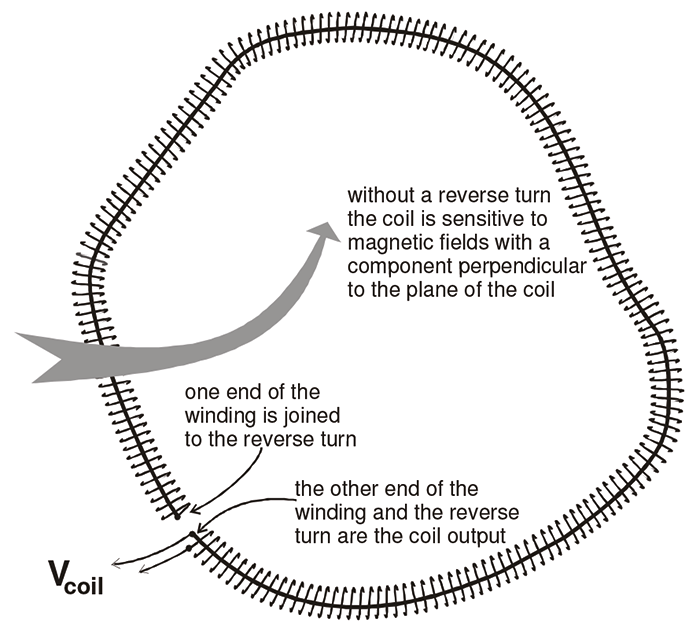Figure 1: Arrangement for the reverse turn

### 3. Analysis

#### 3.1 Basic Theory

The analysis of a long, flexible coil encircling a conductor has been presented in several publications [Ref 1, 2]. Because it is central to the main argument of this Note it is repeated here, in Appendix 1, in a different form than is usual.

The position of the coil is described in cylindrical co-ordinates. The co-ordinates of the ends of the coil areand. Appendix 1 shows that the mutual inductance, M, between the coil and the conductor depends only on q1 and q2 and is given by:where A is the cross-sectional area of the winding and n is the turns density. It introduces the concept of the 'Strength', S, of a winding where:The analysis makes no assumptions about the shape of the coil i.e whether it is circular or not, or whether the ends are in the same plane perpendicular to the Z axis (Fig 2).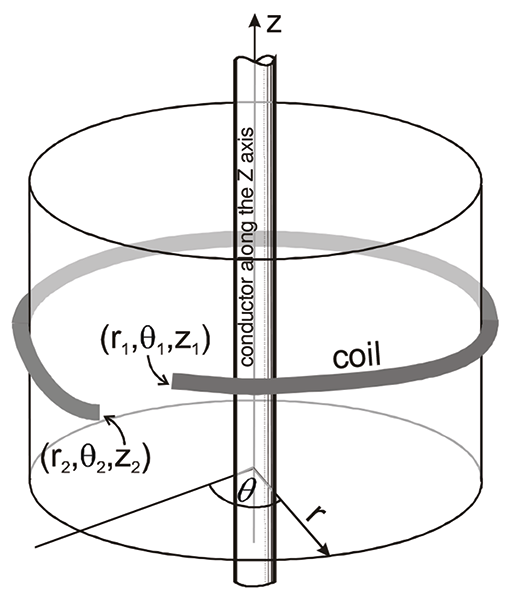Figure 2: Coordinate system

The analysis makes no assumptions about the shape of the coil i.e whether it is circular or not, or whether the ends are in the same plane perpendicular to the Z axis (Fig 2).

Equation 1 shows that the mutual inductance between a Rogowski coil winding and a conductor depends only on the 'Strength' of the winding and the angle subtended at the conductor by the ends of the winding.

#### 3.2 Coil Close to the Conductor

It is shown in Appendix 1 that there can be errors in the analysis in cases where the coil is very close to the conductor. However, provided the distance from the conductor is more than about 7.5 x the cross-sectional diameter of the coil former, the error is small (<0.1%). If the distance is 2.5 x the diameter, the error is about 1%.

Although there can be measurement errors if the coil is close to the conductor there are a number of practical aspects which should be considered. (a) If a Rogowski coil is positioned off-centre from the conductor it does not matter if a short length of the coil is close to the conductor provided that most of the length is at a 'safe' distance. (b) In some configurations a Rogowski coil can be wrapped tightly round a small conductor for the whole of its length. In this situation it is better to calibrate the coil in position. (c) For a large-diameter conductor such as a circular tube, the magnetic fields at the surface of the tube are equivalent to those from a 'thin' conductor along the centre of the tube. Consequently, even if a Rogowski coil is wrapped closely round the tube the errors will be small.

### 4. Examples

This section describes some examples to illustrate how the conclusion of Section 3.1, which relates the mutual inductance to the angle subtended by the ends of the coil at the conductor, can be applied. All the examples are concerned with a coil having a uniform winding in a plane which is perpendicular to the current-carrying conductor.

The examples describe the effect of a gap in the winding on an otherwise perfect coil. This is an idealised situation because in a well-made coil the gap between the ends of the winding can be compensated in some way. This means that the effective gap between the ends is small and no more significant than other defects such as non-uniformities in the cross-section of the coil former. It will be shown in a later Note that other types of defect can be treated in a similar way to a winding gap. In the figures the winding gaps are shown as quite large. This is just for illustrative purposes.

#### 4.1 Uniform Coil with No Gap Between the Ends

The coil is shown as circular for convenience. The same general principle applies for a coil of any shape because the response is determined only by the position of the ends in relation to the conductor.For a conductor at the centre of the coil (Fig 3a) or at any position within the coil (Fig 3b), the angle subtendedisand from Equation 1. For a conductor outside the coil (Fig 3c) the angle subtended is zero and the mutual inductance is zero.

This trivial example illustrates the ideal for a Rogowski coil where the output does not depend on the position of the conductor within the coil and is zero for a conductor outside the coil. Since magnetic fields are caused by electric currents this means that the coil will not be affected by magnetic fields from any external source.

#### 4.2 Uniform Coil with a Gap in the Winding

A gap in the winding or between the coil ends represents a basic winding defect. This situation is illustrated in Figures 4a to 4c which show a circular coil with radius, r. The gap subtends an angleat the conductor when it is at the centre of the coil. For simplicity it is assumed that the angle is 'small' so that sin. Figures 4a to 4c show three different conductor positions.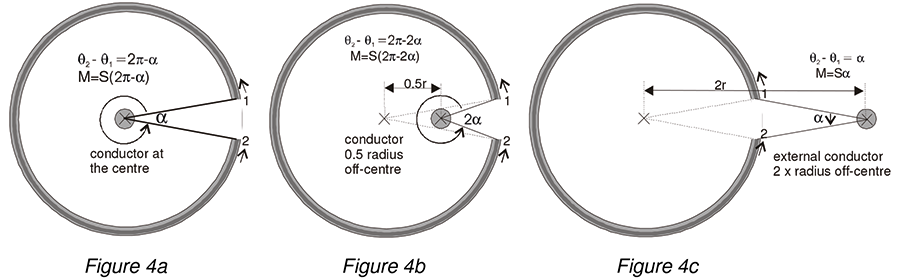Figure Conductor position Angle subtended Mutual inductance
4a central
external: 2 x coil radius $\alpha$

Table 1: Mutual inductance vs Conductor position

By comparing the results from Equations a and b it is seen that the change in mutual inductance which occurs when the conductor is moved 0.5 x r towards the gap is. This is the same as the mutual inductance due to an external conductor at a distance of 2 x r from the centre of the coil (Eq c).

Figure 4d shows the situation where the conductor is offset to the perimeter of the coil (position A). For this case the angle subtended is. This follows directly from the geometric properties of a circle. It is only approximate because it assumes that the conductor is exactly at the perimeter and this is not possible because of the finite size of the conductor. From geometrical considerations the angle subtended is approximatelyfor any position on the circumference (e.g. position B).

It follows that the change in mutual inductance which occurs when the conductor is moved to any point on the perimeter of the coil is approximately half the mutual inductance due to an external conductor at a distance of 2 x r from the centre of the coil (Fig 4c).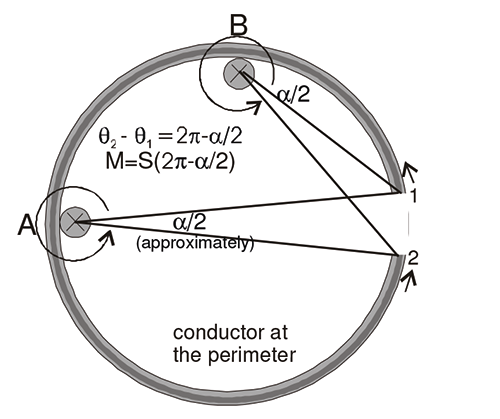Figure 4d: Conductor offset to the perimeter of the coil

#### 4.2.1 Pick-up testing

The results illustrated in Figures 4a to 4d are useful because they show that there is a relationship between the pick-up from an external conductor and the effect of a non-central conductor. This forms the basis of a 'pick-up' test which is used as a quality check on the uniformity of a coil winding.

For a pick-up test the coil is placed near an external conductor at a standard distance of 2 coil radii from the centre of the coil (Fig 4c) and the output voltage is measured. The coil is rotated to find the maximum voltage. This voltage is compared with the voltage from the coil when it is encircling the conductor and gives a ratio which characterises the uniformity of the winding. This single measurement characterises not only the ability of the coil to reject external magnetic fields but also the degree to which the coil is sensitive to currents which are off-centre.

#### 4.3 Coil which is Badly Aligned

Most flexible coils have some kind of end-fitting to ensure that the ends are correctly aligned when the coil is mounted round the conductor. The coil is initially calibrated with the ends correctly aligned (i.e no gap) but if the coil is mounted incorrectly there could be an unintended gap between the ends (Fig 5).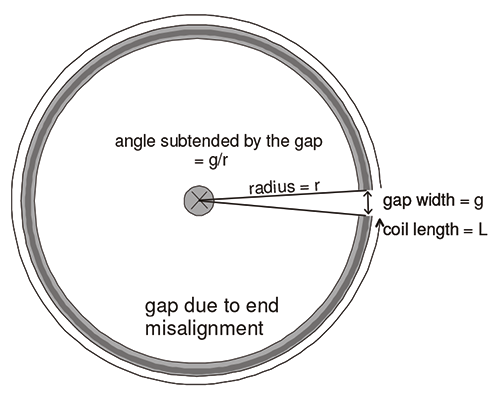Figure 5: Coil with a small gap

If the size of the gap is g, the angle subtended at a conductor placed at the centre of the coil is g/r where r is the radius of the coil. The angle subtended by the coil is approximately

.

Error introduced by the gap =$\frac{}{}$

whereis the length of the coil.

Equation 3 is a simple (and rather obvious) result. We can define a quantity, g%, which is the gap width expressed as a percentage of the total length of the coil. Referring to Figures 4a - 4c gives:

• measurement error due to a misalignment gap = g%
• additional error moving the conductor 0.5 x radius towards the gap = g%
• external pick-up from a conductor 2 x radius from the coil = g%

As a concrete example, for a typical coil length of 1000mm the gap due to misalignment can be as much as 10mm before the error exceeds 1%. This contrasts with the behaviour of a 'split' current transformer where a very small gap between the ends can have a large effect on the accuracy.

#### 4.4.1 Circular coil

A coil with no gap, which is mounted on a rectangular bus-bar, would not be affected by the shape of the conductor. This section considers the case where the coil has a winding gap, g. The bus-bar is modelled as five discrete conductors. In this example it is assumed that each conductor carries the same current but it would be a simple modification to weight the currents in the individual conductors to reflect a non-uniform current distribution and to use more conductors if necessary.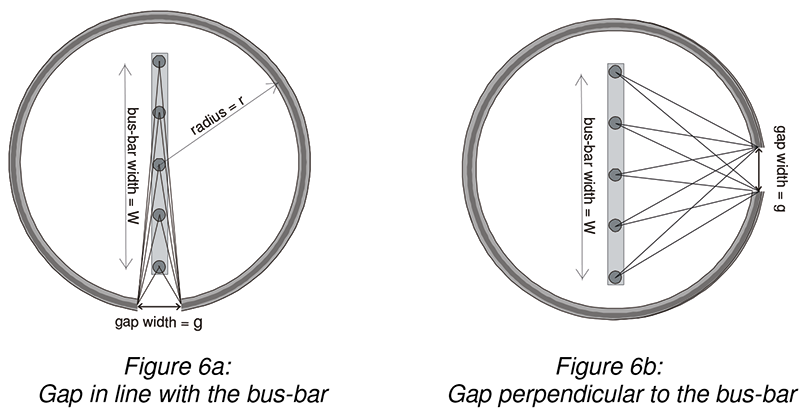Figures 6a and 6b show the arrangement. The coil has a radius, r, and the width of the bus-bar is W. The mutual inductance between the coil and the bus-bar is compared to the mutual inductance if all the current were in a single conductor at the centre of the coil. Two cases are considered: with the gap in line with the bus-bar (Fig 6a), and with the gap perpendidular to the bus-bar (Fig 6b).

Table 2 shows calculated mutual inductances for the following:
circular coil, diameter = 500mm / gap width = 10mm / bus-bar width = 100 - 450mm

The mutual inductances are normalised to the mutual inductance when the current is carried by a single conductor at the centre of the coil.

Bus-bar width W mm Ratio to diameter W/2r Mutual inductance in-line gap Mutual inductance perpendicular gap
100 0.2 0.9999 (-0.01%) 1.0001 (0.01%)
200 0.4 0.9994 (-0.06%) 1.0005 (0.05%)
300 0.6 0.9983 (-0.17%) 1.0009 (0.09%)
400 0.8 0.9950 (-0.50%) 1.0014 (0.14%)
450 0.9 0.9886 (-1.14%) 1.0016 (0.16%)

Table 2: Mutual Inductance to a bus-bar compared with a conductor at the centre of a coil

Table 2 shows that, for a circular coil, the effect of the current being distributed in a bus-bar is small especially for the case where the gap is perpendicular to the bus-bar (Fig 6b). The percentage errors given in the table are proportional to the gap width and for a well-made coil the gap could be considerably less than 10mm.

For the perpendicular case, the mutual inductance is higher when the current is distributed in a bus-bar, than when the current is concentrated in the centre. This can be seen qualitatively by considering the angles. Currents flowing at the centre of the coil subtend a larger angle at the gap than currents off-centre. The mutual inductance is determined by the average of all the angles.

#### 4.4.2 Race-track' coil

In practice, especially with large bus-bars and when there is another bus-bar adjacent, it is convenient to use a non-circular coil, described as 'race-track' shape.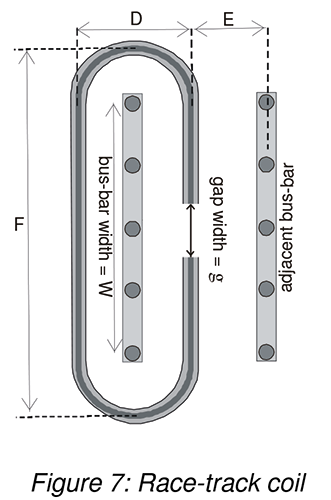Figure 7 shows the arrangement. The coil has a long dimension, F, and a short dimension D. Table 3 shows calculated mutual inductances for
the following:

Long dimension (F) = 500mm / gap width = 10mm / bus-bar width
(W) = 400mm / Short dimension (D) = 100 - 500mm.

Mutual inductances are normalised to the mutual inductance when the current is carried by a single conductor at the centre of a circular coil, diameter 500mm with the same gap. The numbers for an in-line gap are the same as for a circular coil with diameter 500mm (see Fig 6a and Table 2).

short dimension D mm mutual inductance in-line gap mutual inductance perpendicular gap
500 0.9950 (-0.50%) 1.0014 (0.14%)
400 0.9950 (-0.50%) 1.0006 (0.06%)
300 0.9950 (-0.50%) 0.9998 (-0.02%)
200 0.9950 (-0.50%) 0.9987 (-0.13%)
100 0.9950 (-0.50%) 0.9967 (-0.33%)

Table 3: Mutual inductance to a bus-bar compared with a conductor at the centre of a circular coil

Table 3 shows that, for a race-track coil, the errors are low even for a narrow coil when the coil gap is close to the bus-bar. This is because the currents flowing at the edges of the bus-bar make a very low angle at the gap.

#### 4.4.3 Pick-up from an adjacent bus-bar

It is also possible to calculate the pick-up from the adjacent bus-bar which is at a distance, E, from the gap (Fig 7).

Adjacent bus-bar width = 400mm / gap = 10mm / distance from the gap (E) = 400- 50mm

Table 4 shows the mutual inductance (pick-up) between the race-track coil and the adjacent bus-bar for different values of E, normalised as previously. The gap width, g, used in the calculation is 10mm. For a smaller gap the pick-up values would be smaller in proportion.

Distance from the gap, E (mm) Pick-up
400 0.36%
300 0.45%
200 0.58%
100 0.77%
50 0.97%

Table 4: Mutual inductance to an adjacent bus-bar (pick-up) compared with a conductor at the centre of a circular coil

The results in Table 4 show that, although the distance to the adjacent bus-bar changes by a factor of 8, the effect on the pick-up is much smaller. This can be seen qualitatively by considering the angles subtended at the gap. When the distance between the adjacent bus-bar and the gap becomes smaller, the angle, subtended at the gap by currents near the centre of the bus-bar, increases causing an increase in pick-up. However, for currents at the edges of the bus-bar the opposite is the case and the angles subtended become smaller.

## 5. Experimental Checks

The mutual inductance of a coil to a conductor was measured in a variety of situations using a coil with a gap and the conductor either threading the coil or external to the coil.

#### 5.1 Mutual Inductance Measurements

The measurements were made using the calibration facilities developed by Rocoil. The current was provided in a rectangular current loop 800mm x 1500mm. The measuring position was in the middle of one of the long sides of the current loop. The rectangular loop consisted of 50 turns of wire, thus a current of 5A in the wire was equivalent to 250A in the loop. This current was supplied by a power amplifier which enabled a high level of control of both current and frequency. The current was measured using precision resistors which had traceable calibrations.

Mutual inductance was measured by comparing the voltage output of the coil with the voltage measured across the precision resistor. These two voltages were measured directly as a ratio which eliminated any errors caused by a drift in the current. Two methods were used to make this comparison.

1. Voltmeter Method: The two voltages are measured simultaneously on voltmeters and the ratio is computed directly. The result is divided by the frequency to take into account that the output of a Rogowski coil is proportional to frequency. The measurement is made at a frequency different from the mains frequency, and the voltmeters are frequency selective. This minimises errors due to mains
interference.
2. Bridge Method: A Mutual Inductance Bridge, developed by Rocoil, uses a standard integrator to integrate the coil output. The output of the integrator is compared directly with the voltage across the standard resistor.

Both of these calibration methods have been in use for many years and are regularly maintained and checked. They agree with each other to 1 part in 10,000 and the overall uncertainty is estimated to be less than 0.1%

#### 5.2 Off-centre Mutual Inductance

The measurements were made using a length of flexible coil wound on a silicone rubber former with diameter 7.1mm. It was close-wound (adjacent turns touching) in a single layer using 0.14mm enamel-insulated copper wire.

Close-winding is a good method to get a uniform winding. The coil was connected using a reverse turn as shown in Figure 1. There was a substantial copper core along the centre of the coil former which, in addition to serving as a reverse turn, also prevented the silicone-rubber from stretching lengthwise.

The coil was bent into a loop with zero gap between the ends and the mutual inductance was measured to be.

This mutual inductance measurement enabled the Strength of the winding to be determined. Referring to Equation A7, the strength of the winding is given by: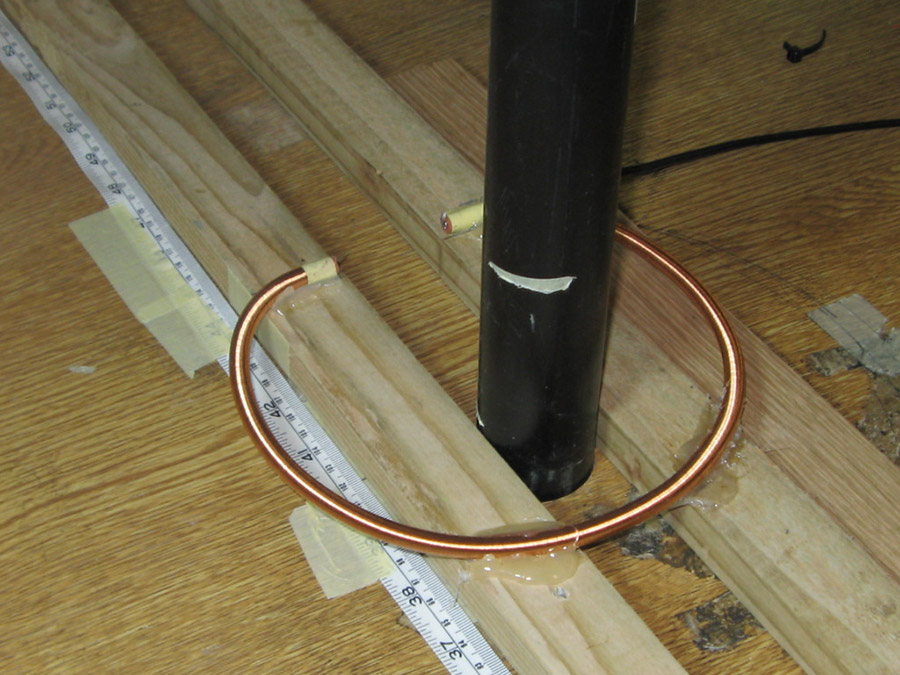Figure 8: Experimental arrangement

The coil was then bent into an approximately circular shape and mounted on a wooden framework with a gap of 50mm between the ends as shown in Figure 8. The wooden frame enabled the coil to be moved accurately relative to the conductor. The position with the conductor central in the gap was used as the zero reference. Measurements were made moving the coil at 10mm intervals from -130mm (conductor inside the coil) to +160mm (conductor outside the coil).Figure 9: Conductor positions

At each position the mutual inductance between the coil and the conductor was measured.

The mutual inductance at each position was also calculated by multiplying the angle subtended at the conductor by the strength of the winding.

The calculation also included the contribution from the return conductor in the current loop which was a distance of 800mm. This contribution was extremely small.

The results are presented in the graph (Fig 10). The red line shows the mutual inductance calculated from angles. The markers show the measured values. The agreement is very good over most of the range except for a difference of about 1% when the conductor was inside the coil close to the part of the coil opposite the gap. This is attributed to a small non-uniformity in the coil winding.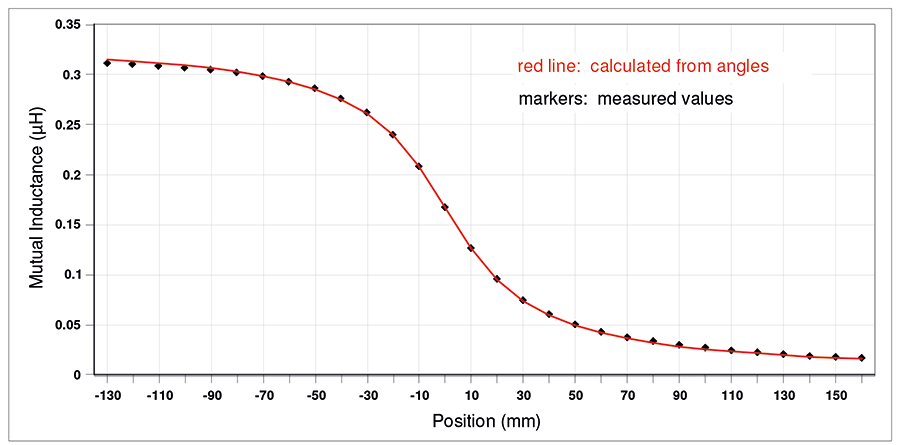Figure 10: Comparison between measured and calculated results

#### 5.3 External Pick-up

A second test was carried out to determine the effect of an external conductor on a coil with a gap in the winding. The coil material used had a similar specification to that described in Section 5.2. The coil was connected up using a reverse turn as shown in Figure 1. A length of flexible coil was mounted on a turntable with a gap of 40mm between the ends. A 40mm gap is much larger than would be expected in real life, even with a badly-constructed coil, but a large gap was chosen to give a large signal that could be measured reasonably accurately and not be influenced too significantly by imperfections in the coil winding.

The coil was mounted close to a conductor as shown in Figure 11. It was rotated at 20° intervals and the mutual inductance between the coil and the conductor was measured. As in the previous example, the mutual inductance at each position was also calculated by multiplying the angle subtended by the conductor at the gap by the Strength of the winding. The calculation also included the contribution from the return conductor in the current loop which was a distance of 800mm. In this case the contribution of the return conductor was significant.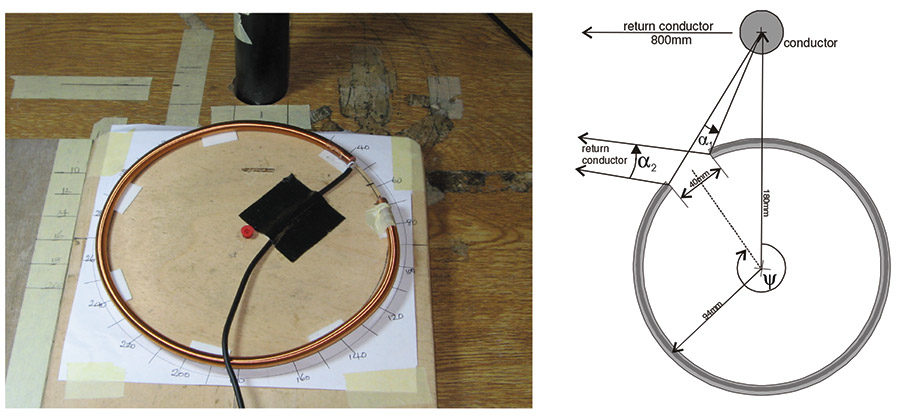Figure 11: Arrangement for external pick-up measurements

The results are presented in the graph (Fig 12). The red line shows the mutual inductance calculated from angles. The markers show the measured values. Zero angle corresponds to the angular position with the gap closest to the conductor. The results are compared with the mutual inductance of the coil when it is encircling the conductor and expressed as a percentage. The worst case difference between the calculated and measured values is less than 0.5%. This could be explained by imperfections in the coil winding, which is why the measurements were made using a large gap. The asymmetry in the results shows the influence of the return conductor in these measurements.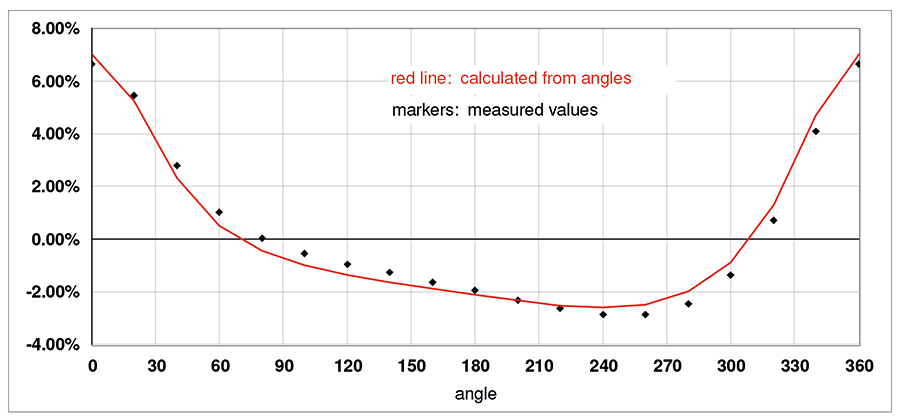Figure 12: External pick-up measurements

### 6. Conclusions

1) The mutual inductance between a thin, uniformly wound Rogowswki coil and a conductor is proportional to the angle subtended at the conductor by the ends of the winding.

2) From Section 3.2, a coil can be considered thin if the distance from the conductor is more than 2.5 x the cross-sectional diameter of the coil (1% accuracy) or 7.5 x the cross-sectional diameter of the coil (0.1% accuracy).

3) The result in conclusion 1 can be used to calculate the effect on the mutual inductance of off-centre conductors, multiple conductors, external conductors and non-circular conductors. The relationship can also be used to make qualitative estimates without the need for mathematical analysis.

4) For a coil with no gap the mutual inductance is not affected by the position of the conductor, and the coil is not affected by external magnetic fields.

5) There is a close relationship between the pick-up from an external conductor and the effect of off-centre conductors on the output from a coil (Section 4.2).

6) Measurement of the pick-up between a coil and an external conductor is a good quality check of the uniformity of the coil winding.

7) The result in Conclusion (1) has been verified experimentally.

### APPENDIX 1

#### A1.1 Basic Analysis

The analysis of a long, flexible coil encircling a conductor has been presented in several publications [Ref 1, 2, 3]. Because it is central to the main argument of this Note it will be repeated here in a different form than is usual.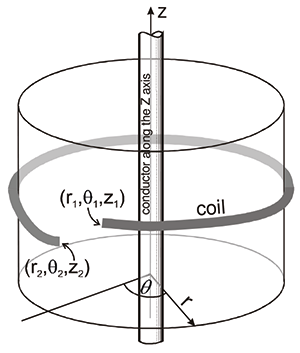The position of the winding is shown in cylindrical coordinates where the current-carrying conductor is along the Z axis. The view on the right is shown looking along the Z axis.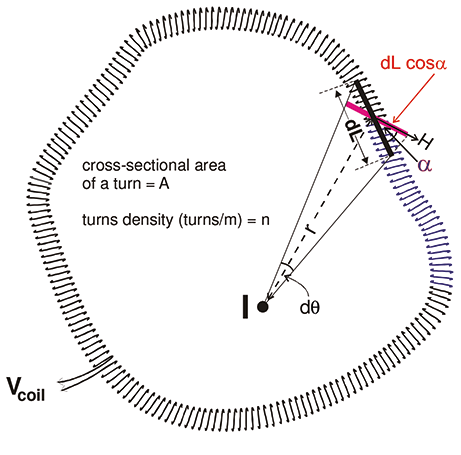Figure A1: Deriving the mutual inductance

Figure A1 shows a long coil encircling a conductor which carries a current, I. The cross-sectional area of the winding is A and the turns density (turns/m) is n.

Consider a section dL of winding. The number of turns in this section is ndL and the area turns is nAdL.

The time-dependent current I(t) threading the coil causes a magnetic field H(t), where(A1)

r is the distance of the section dL from the conductor. The direction of H is perpendicular to the direction of r and is in a plane perpendicular to the direction of the current.

The flux linked to an element dL is given by:(A2)

whereis the angle between the direction of the element dL and the magnetic field H. The element dL need not necessarily be in the plane perpendicular to the current.

The voltage induced in the element is:Substituting from (A1)(A3)

From Figure A1 it is seen thatwhereis the angle subtended by the ends of the element dL at the conductor, measured in the plane perpendicular to the conductor.

Equation A3 becomes:(A4)

Integrating along the coil gives:(A5)

where and are the angular positions at the two ends of the coil.

For a mutual inductance, M, the voltage output from the coil is given by. Hence, the mutual inductance between the coil and the conductor is given by:(A6)

For the special case where the two ends of the coil are coincident (no gap), the mutual inductance reduces to the well-known expression:.

The quantityreflects the output of a section of winding and, for purposes of this Note, is referred to as the 'Strength', S, of the winding. If the mutual inductance of a coil with no gap is known, the
Strength is given by:(A7)

Equation A6 shows that the mutual inductance between the coil and the conductor depends only on the angular positions of the ends and the 'Strength' ,S, of the winding. For cases where the coil is not closed, equation A6 offers a simple and intuitive method of evaluating the mutual inductance between a coil and a conductor, or several conductors, and how it depends on the position of the conductor whether it is inside or outside the loop of the coil.

Equation A6 makes no assumptions about the shape of the coil i.e whether it is circular or not or whether the ends are in the same plane perpendicular to the Z axis (Fig 2).

#### A1.2 The 'Thin Coil' approximation

In the analysis given above the flux threading a turn of wire was calculated as the flux density at the centre of the turn multiplied by the area of the turn (Eq A2). This is actually an approximation because the flux density varies across the area of the turn depending on the distance from the conductor and a more correct procedure would be to integrate the flux density over the area. This approximation means that the analysis is only strictly accurate for coils which have a small cross-sectional area. The geometry is shown in Figure A2.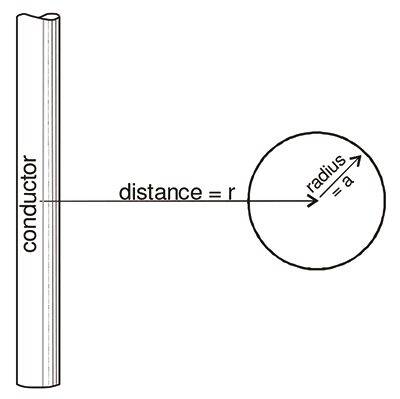Figure A2: Single turn of wire close to a conductor

To determine what is meant by a 'thin coil' in this context the flux threading a circular loop of wire near a conductor was calculated by two methods:

flux(1) by multiplying the flux at the centre of the loop by the area, (approximate flux calculation),
and flux(2) by integrating the flux density over the area of the loop, (full flux calculation).

Table A1 shows the error associated with this approximation. The error depends on the ratio of the distance, r, from the conductor to the cross-sectional diameter, 2a, of the turn. The Table shows that, provided the distance from the conductor is more than about 7.5 x the cross-sectional diameter, the error is small (<0.1%). If the distance is 2.5 times the diameter the error is about 1%.

r/2a flux(2) / flux(1)
25 1.0001
10 1.0006
7.5 1.0011
5 1.0025
2.5 1.0102
2 1.0161

Table A1: Comparison of the flux in a circular loop calculated by two different methods

### References

1. Ward, D. A. and Exon, J. La T.; ‘Using Rogowski coils for Transient Current Measurements’. IEE Engineering Science and Education Journal, June 1993, pp105 - 113.
2. Stoll, R. L.; 'Method of Measuring Alternating Currents Without Disturbing the Conducting Circuit'. IEE Proc. 122, (10), October 1975, pp. 1166 - 1167.
3. Chattock, A. P. 'On a Magnetic Potentiometer', Phil. Mag. Vol. 24, No 5, 1887, p94.
4. Crotti, G., Giordano, D. and Morando, A. 'Analysis of Rogowski Coil Behaviour Under Non-Ideal Measurement Conditions'. XIX IMEKO World Congress Fundamental and Applied Metrology, September 6 - 11, 2009, Lisbon, Portugal.